# 深度学习利器之自动微分(2)

## 0x00 摘要

• 反向传播时的自动梯度计算和更新，也就是自动微分
• 使用 GPU 进行计算。

## 0x01 前情回顾

• 手动求解法(Manual Differentiation) : 完全手动完成，依据链式法则解出梯度公式，带入数值，得到梯度。
• 数值微分法(Numerical Differentiation) ：利用导数的原始定义，直接求解微分值。
• 符号微分法(Symbolic Differentiation) : 利用求导规则对表达式进行自动计算，其计算结果是导函数的表达式而非具体的数值。即，先求解析解，然后转换为程序，再通过程序计算出函数的梯度。
• 自动微分法(Automatic Differentiation) ：介于数值微分和符号微分之间的方法，采用类似有向图的计算来求解微分值。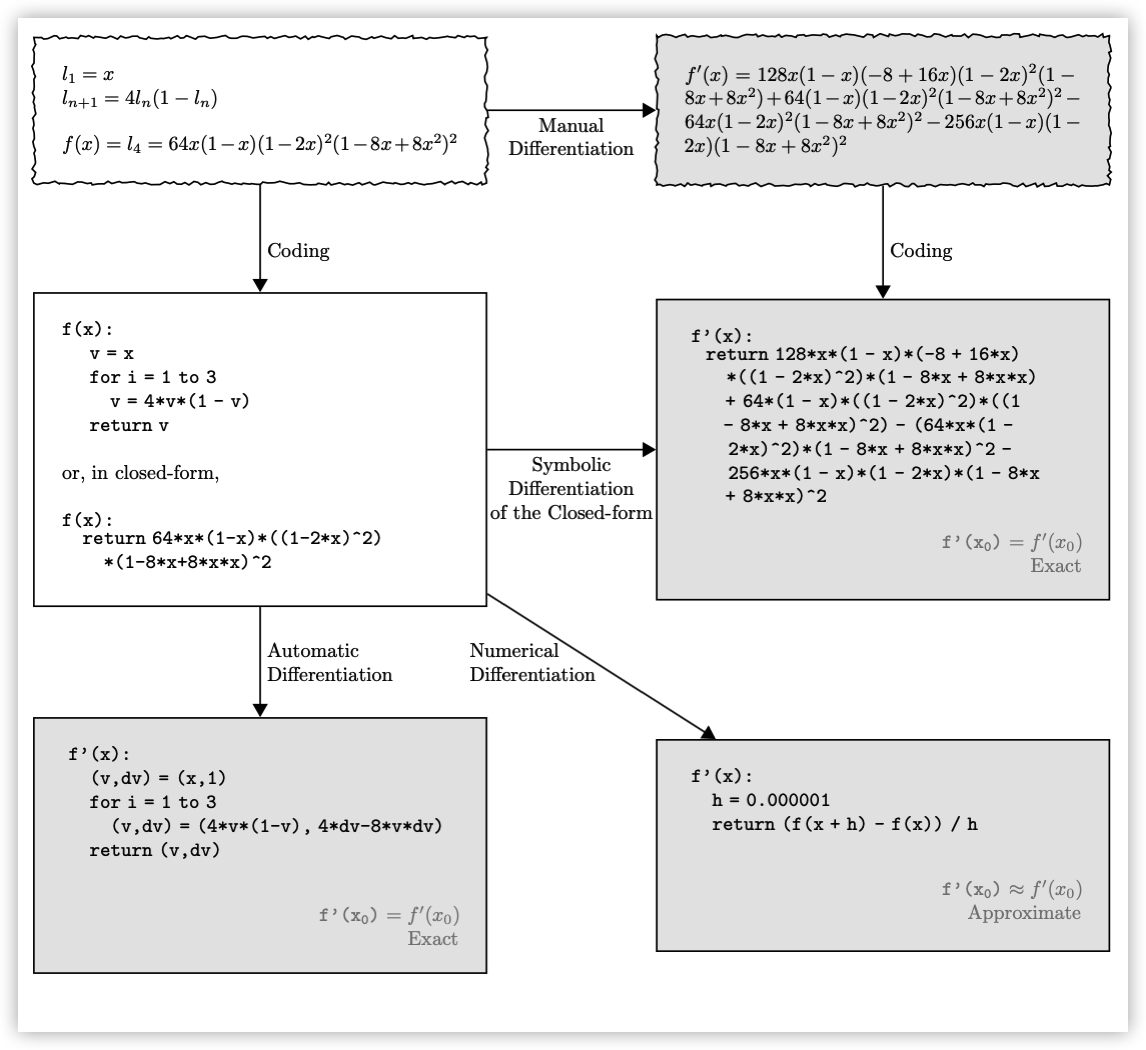## 0x02 自动微分

### 2.1 分解计算

$y=f(g(h(x)))=f(g(h(w_0)))=f(g(w_1))=f(w_2)=w_3 \\$

\begin{align} y=f(g(h(x)))=f(g(h(w_0)))=f(g(w_1))=f(w_2)=w_3 \\ w_0=x\\ w_1=h(w_0)\\ w_2=g(w_1)\\ w_3=f(w_2)=y \end{align}

### 2.2 计算模式

• 前向自动微分（Forward Automatic Differentiation，也叫做 tangent mode AD）或者前向累积梯度（前向模式）。

$\frac{dw_i}{dx}=\frac{dw_i}{dw_{i-1}}\frac{dw_{i-1}}{dx} \qquad with\ w_3=y$

$\frac{dy}{dw_i} = \frac{dy}{dw_{i+1}}\frac{dw_{i+1}}{dw_i} \qquad with \ w_0 = x$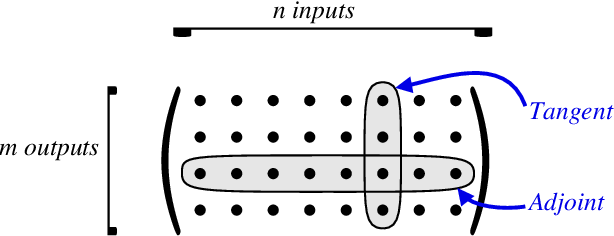$\frac{∂x^k}{∂x^0_j} = \frac{∂x^k}{∂x^{k-1}} \frac{∂x^{k-1}}{∂x^0_j}$

$\frac{∂x^L_i}{∂x^k} = \frac{∂x^L_i}{∂x^{k+1}} \frac{∂x^{k+1}}{∂x^k}$

### 2.3 样例

• 输入变量 ：自变量维度为 n，这里 n = 2，输入变量就是 $x_1, x_2$

• 中间变量 ：中间变量这里是 $v_{-1}$ 到 $v_5$，在计算过程中，只需要针对这些中间变量做处理即可：将符号微分法应用于最基本的算子，然后代入数值，保留中间结果，最后再应用于整个函数。

• 输出变量 ：假设输出变量维度为 m，这里 m = 1，输出变量就是 $y_1$，也就是$f(x_1, x_2)$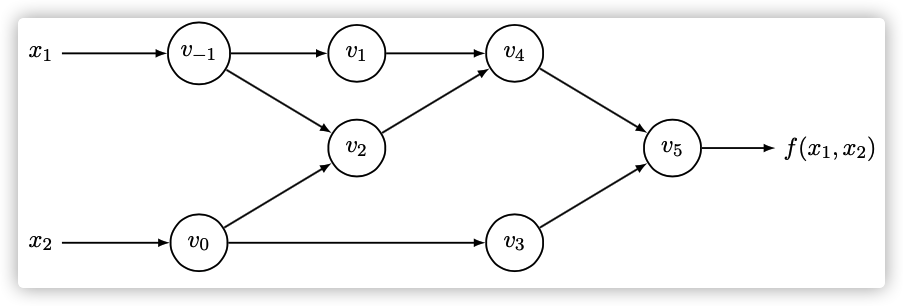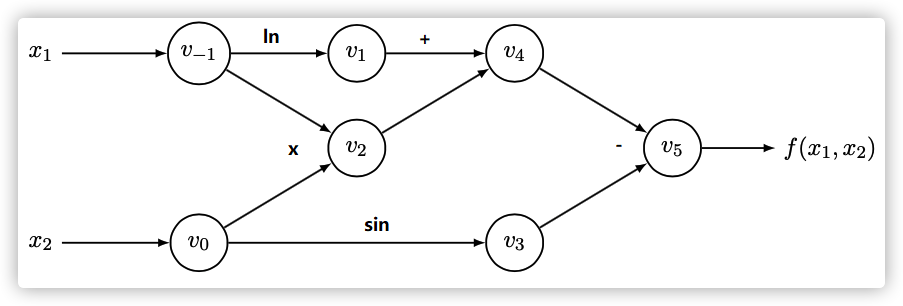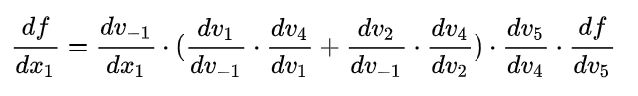• 将这个式子从前往后算的叫前向模式（Forward Mode）。
• 将这个式子从后往前算的叫逆向模式（Reverse Mode）。

### 2.4 前向模式（Forward Mode）

#### 2.4.1 计算过程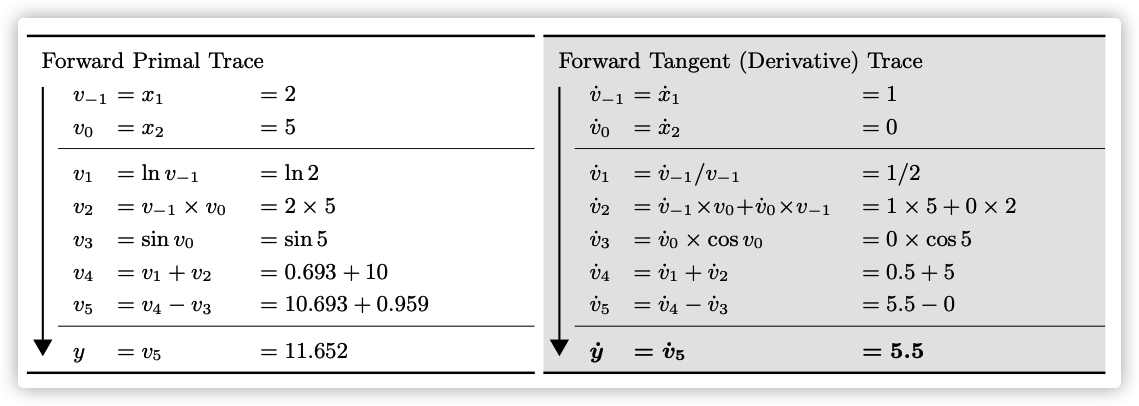1. 我们给输入节点赋值：$v_{-1} = x_1 = 2$$v_{0} = x_2 = 5$
2. 计算$v_1$节点：$v_1 = ln\ v_{-1} = ln\ x_1 = ln\ 2$
3. 计算 $v_2$ 节点，节点 v_2 依赖于$v_{-1}$$v_0$，即: $v_2=v_{−1} \times v_0=x_1 \times x_2= 2 \times 5 = 10$
4. 计算 v3 节点，$v_3 = sin \ v_0 = sin \ 5$
5. 计算 $v_4 $节点，$v_4 = v_1 + v_2 = 0.693 + 10$
6. 计算 $v_5$节点，$v_5 = v_1 + v_2 = 10.693 + 0.959$
7. 最终 $y = v_5 = 11.652$

1. $v_{-1}$ 节点对于$x_1$梯度 : 因为 $v_{-1} = x_1$，所以 $\frac{∂v_{-1}}{∂x{1}} = 1$

2. $v_{0}$ 节点对于$x_1$梯度 : 因为 $v_{0} = x_2$，这样就和 $x_{1}$ 无关，所以 $\frac{∂v_{0}}{∂x{1}} = 0$

3. $v_{1}$ 节点对于$x_1$梯度 : $\frac{∂v_{1}}{∂x_{1}} = \frac{∂\ log \ x_1}{∂x_1} = \frac{1}{x_1} = \frac{1}{2}$

4. $v_{2}$ 节点对于$x_1$梯度 : $\frac{∂v_{2}}{∂x_{1}} = \frac{∂v_{-1} }{∂x_1}\times v_0 + \frac{∂v_{0}}{ ∂x_1}\times v_{-1} = 1 \times 5 + 0 \times 2$

5. $v_{3}$ 节点对于$x_1$梯度 : $\frac{∂v_{0}}{∂x_{1}} \times cos \ v_0 = 0 \times cos \ 5$

6. $v_{4}$ 节点对于$x_1$梯度 : $\frac{∂v_{1}}{∂x_{1}} = \frac{∂ v_1}{∂x_1} + \frac{∂ v_2}{∂x_1} = 0.5 + 5$

7. $v_{5}$ 节点对于$x_1$梯度 : $\frac{∂v_{1}}{∂x_{1}} = \frac{∂v_4}{∂x_1} - \frac{∂v_3}{∂x_1} = 5.5 - 0$

8. 所以 $\frac{∂y}{∂x_{1}} = \frac{∂v_5}{∂x_1} = 5.5$

• 计算图各个节点的数值和该节点的导数可同步求出。因为我们已经了解了深度学习，所以从深度学习角度来看，前向模式只需要完成计算出计算图中各节点的值。求导数则可以由反向模式来实现
• 注意到每一步的求导都会利用到上一步的求导结果，这样不至于重复计算，因此也不会产生像符号微分法的"expression swell"问题。自动微分的前向模式实际上与我们在微积分里所学的求导过程一致。

#### 3.4.2 推广

$f : R ^n \rightarrow R^m$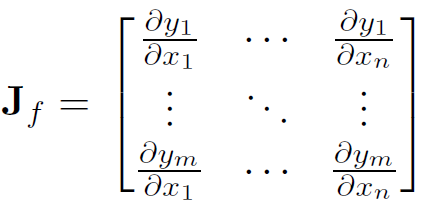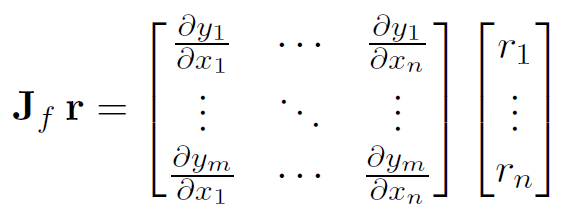#### 3.4.3 问题

• 每次前向计算只能计算对一个自变量的偏导数，对于一元函数求导是高效的。但是机器学习模型的参数通常有 $10^6$数量级之多。
• 如果有一个函数，其输入有 n 个，输出有 m个，对于每个输入来说，前向模式都需要遍历计算过程以得到这个输入的导数，求解整个函数梯度需要 n 遍如上计算过程。尤其是神经网络这种 $n \gg m$ 的模型，前向模式就太低效了，重复遍历计算过程 $10^6$次显然是无法接受的。

### 3.5 反向模式（Reverse Mode）

#### 3.5.1 思路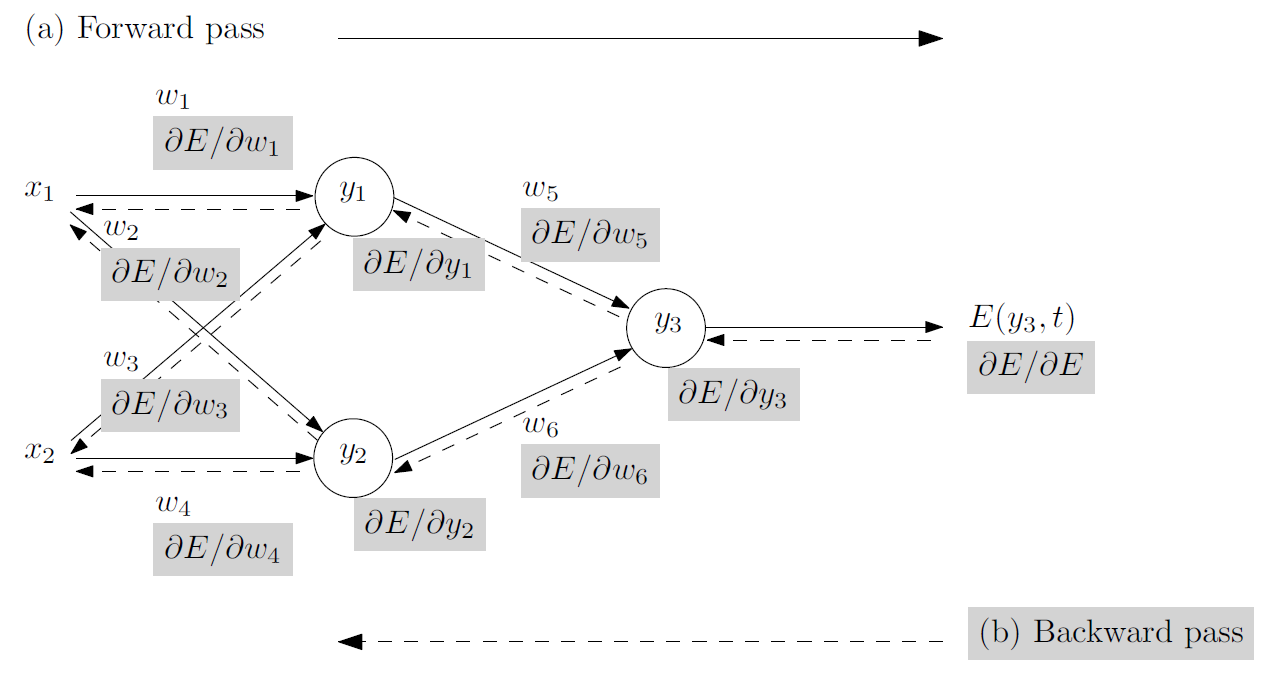#### 3.5.2 计算过程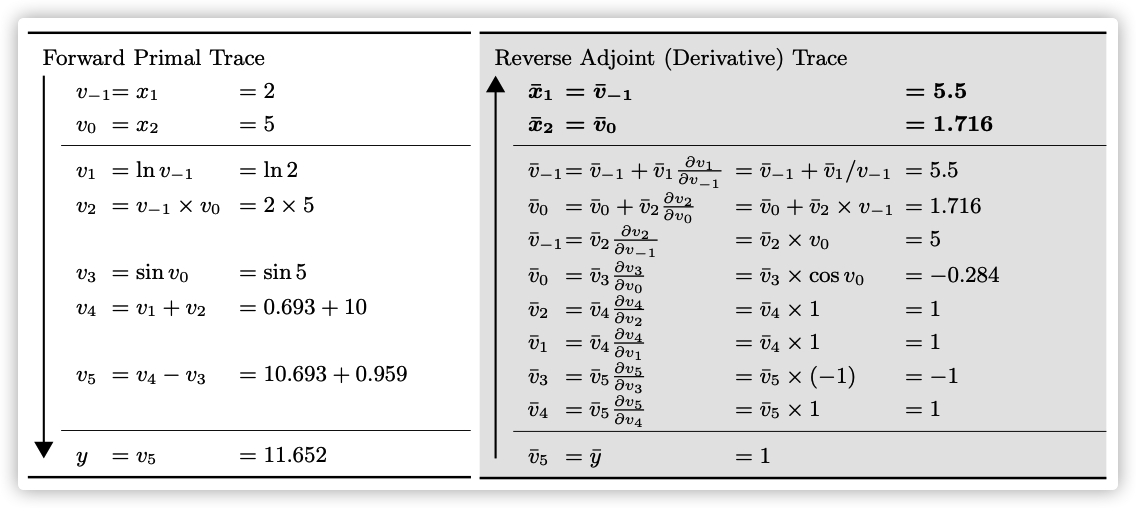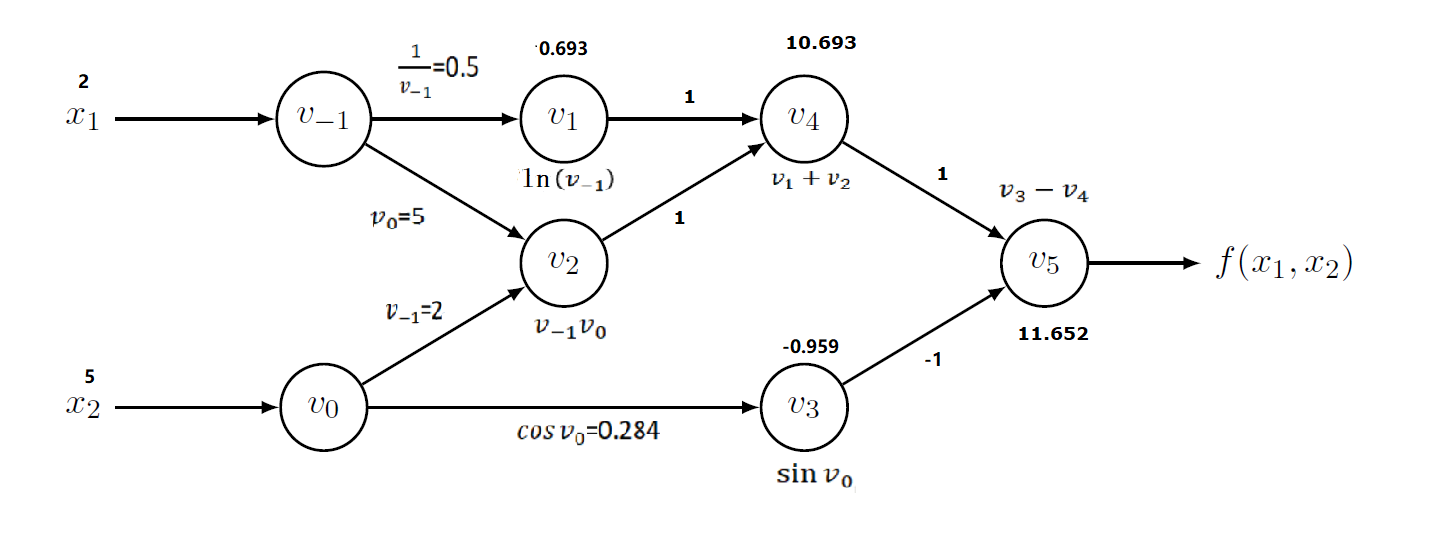1. 计算 y 对 $v_5$的导数值，因为 $y = v_5$, 所以 $\frac{∂y}{∂v_5} = v'_5 = 1$

2. 计算 y 对 $v_4$的导数值，因为 $v_4$ 在图上只有一个后续节点 $v_5$, 并且 $v_5=v_3−v_4$，所以依据链式法则得到$\frac{∂y}{∂v_4} =\frac{∂y}{∂v5} \times \frac{∂v5}{∂v4} = v'_5 \frac{∂v_5}{∂v_4} = v'_5 \frac{∂(v_3 - v_4)}{∂v_4} = v'_5 \times 1 = 1$，将结果写在$v_4$指向$v_5$的边上。

3. 计算 y 对 $v_3$的导数值，因为 $v_3$ 在图上只有一个后续节点 $v_5$, 并且 $v_5=v_3−v_4$，所以依据链式法则得到$\frac{∂y}{∂v_3} =\frac{∂y}{∂v5} \times \frac{∂v5}{∂v3} = v'_5 \frac{∂v_5}{∂v_3} = v'_5 \frac{∂(v_3 - v_4)}{∂v_3} = v'_5 \times (-1) = -1$，，将结果写在$v_3$指向$v_5$的边上。

4. 计算 y 对 $v_1$的导数值，因为 $v_1$ 在图上只有一个后续节点 $v_4$, 并且 $v_4=v_1+v_2$，所以依据链式法则得到$\frac{∂y}{∂v_1} =\frac{∂y}{∂v_1} \times \frac{∂v_4}{∂v_1} = v'_4 \frac{∂v_4}{∂v_1} = v'_4 \frac{∂(v_1 + v_1)}{∂v_1} = v'_4 \times 1 = 1$，，将结果写在$v_1$指向$v_4$的边上。

5. 计算 y 对 $v_2$的导数值，因为 $v_2$ 在图上只有一个后续节点 $v_4$, 并且 $v_4=v_1+v_2$，所以依据链式法则得到$\frac{∂y}{∂v_2} =\frac{∂y}{∂v_4} \times \frac{∂v_4}{∂v_2} = v'_4 \frac{∂v_4}{∂v_2} = v'_4 \frac{∂(v_1 + v_2)}{∂v_2} = v'_4 \times 1 = 1$，，将结果写在$v_2$指向$v_4$的边上。

6. 接下来要计算 y 对 $v_0$的导数值 和 y 对 $v_{-1}$的导数值，因为 $v_0$$v_{-1}$ 都是后续有两个节点，

1. $v_0$ 在图上有两个后续节点 $v_2$$v_3$, 并且 $v_2=v_{-1} \times v_0$$v_3= sin \ v_0$
2. $v_{-1}$ 在图上有两个后续节点 $v_2$$v_1$, 并且 $v_2=v_{-1} \times v_0$$v_1= ln \ v_{-1}$

所以我们只能分开计算。

7. 计算 $\frac{∂v_3}{∂v_0} = \frac{∂sin\ v_0}{∂v_0} = cos\ v_0 = 0.284$，将结果写在$v_0$指向$v_3$的边上。

8. 计算 $\frac{∂v_2}{∂v_0} = \frac{∂(v_{-1}v_0)}{∂v_0} = v_{-1} = 2$，将结果写在$v_0$指向$v_2$的边上。

9. 计算 $\frac{∂v_2}{∂v_{-1}} = \frac{∂(v_{-1}v_0)}{∂v_{-1}} = v_0 = 5$，将结果写在$v_{-1}$指向$v_2$的边上。

10. 计算 $\frac{∂v_1}{∂v_{-1}} = \frac{∂(ln \ v_{-1})}{∂v_{-1}} = \frac{1}{x_1} = 0.5$，将结果写在$v_{-1}$指向$v_1$的边上。

• $v_5→v_4→v_1→v_{−1}$ ：其数值乘积是 1∗1∗0.5=0.5。
• $v_5→v_4→v_2→v_{−1}$ ：其数值乘积是 1∗1∗ 5= 5。

• $v_5→v_4→v_2→v_0$ ：其数值乘积是 1∗1∗2=2.0。
• $v_5→v_3→v_0$ ：其数值乘积是 (−1)∗0.284=−0.284。

#### 3.5.3 推广

$f : R ^n \rightarrow R^m$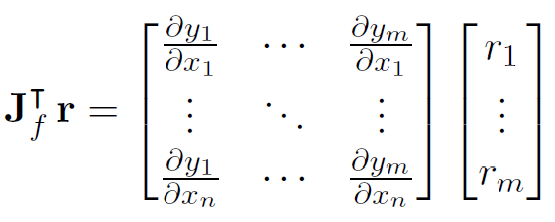#### 3.5.4 内存问题和inplace 操作

inplace 指的是在不更改变量的内存地址的情况下，直接修改变量的值。

# 情景 1，不是 inplace，类似 Python 中的 i=i+1
a = a.exp()
# 情景 2，是 inplace 操作，类似 i+=1
a = 10


### 3.6 比较## 0x04 PyTorch

### 4.1 原理

• 数学基础：复合函数，链式求导法则和雅克比矩阵；
• 工程基础 ：Tensor 构成的计算图（DAG有向无环图）；

#### 4.1.1 雅克比矩阵

$J = [\frac{∂f}{∂x_1} ... \frac{∂f}{∂x_n}] = \left\{ \begin{matrix} \frac{∂f_1}{∂x_1} & \cdots & \frac{∂f_1}{∂x_n}\\ \vdots & \ddots & \vdots \\ \frac{∂f_m}{∂x_1} & \cdots & \frac{∂f_m}{∂x_n} \end{matrix} \right\}$

$J = \left(\begin{array}{cc} \frac{\partial \bf{y}}{\partial x_{1}} & ... & \frac{\partial \bf{y}}{\partial x_{n}} \end{array}\right) = \left(\begin{array}{ccc} \frac{\partial y_{1}}{\partial x_{1}} & \cdots & \frac{\partial y_{1}}{\partial x_{n}}\\ \vdots & \ddots & \vdots\\ \frac{\partial y_{m}}{\partial x_{1}} & \cdots & \frac{\partial y_{m}}{\partial x_{n}} \end{array}\right)$

##### backward 函数

def backward(self, gradient=None,...)


• 如果 gradient 是向量 vector。PyTorch 不会显式构造整个雅可比矩阵（实际的梯度），而是直接计算 Jacobian 乘积（Jacobian vector product），这通常更简单有效，而且存储真正的梯度会浪费大量空间。

backward 方法最终调用的是torch.autograd 类。在 PyTorch 之中，torch.autograd 类从数学来说就是一个雅可比向量积计算引擎。

$\vec{v} = \left(\begin{array}{ccc}\frac{\partial l}{\partial y_{1}} & \cdots & \frac{\partial l}{\partial y_{m}}\end{array}\right)^{T}$

$J^{T}\cdot \vec{v}=\left(\begin{array}{ccc} \frac{\partial y_{1}}{\partial x_{1}} & \cdots & \frac{\partial y_{m}}{\partial x_{1}}\\ \vdots & \ddots & \vdots\\ \frac{\partial y_{1}}{\partial x_{n}} & \cdots & \frac{\partial y_{m}}{\partial x_{n}} \end{array}\right)\left(\begin{array}{c} \frac{\partial l}{\partial y_{1}}\\ \vdots\\ \frac{\partial l}{\partial y_{m}} \end{array}\right)=\left(\begin{array}{c} \frac{\partial l}{\partial x_{1}}\\ \vdots\\ \frac{\partial l}{\partial x_{n}} \end{array}\right)$

#### 4.1.2 计算图

• 原始函数建立计算图，将问题转化成一种有向无环图。
• 进行正向传播，计算出中间节点，并记录计算图中的节点依赖关系。
• 反向传播，从输出开始遍历计算图，计算输出对于每个节点的导数。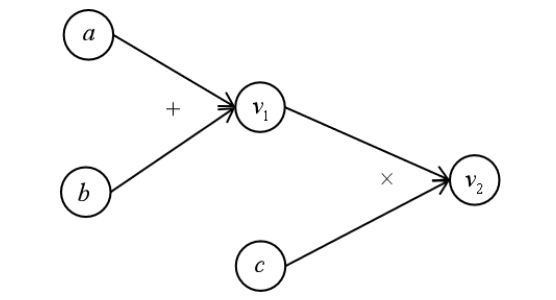#### 4.1.3 神经网络中的链式法则

$b=w_1∗a \\ c=w_2∗a\\ d=w_3∗b+w_4∗c\\ L=10−d$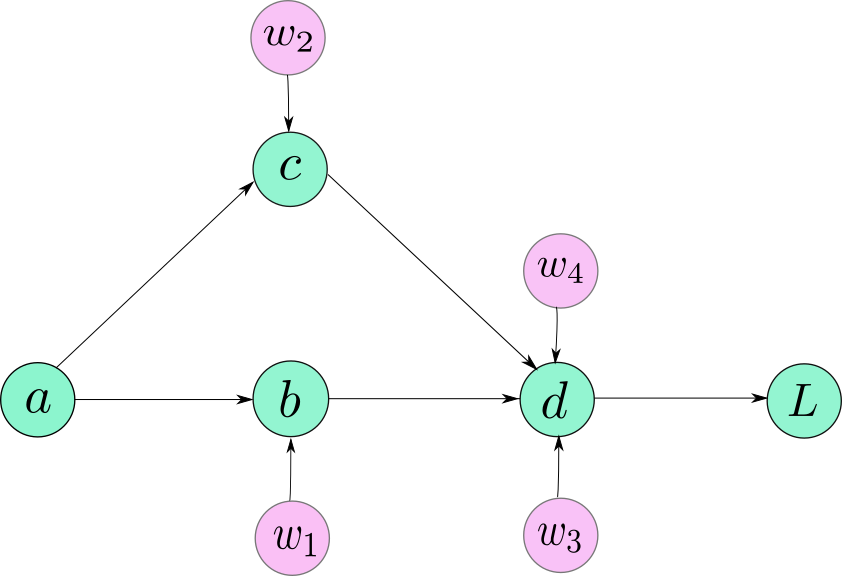$\frac{∂L}{∂w_4} = \frac{\partial{L}}{\partial{d}} * \frac{\partial{d}}{\partial{w_4}}\\ \frac{\partial{L}}{\partial{w_3}} = \frac{\partial{L}}{\partial{d}} * \frac{\partial{d}}{\partial{w_3}} \\ \frac{\partial{L}}{\partial{w_2}} = \frac{\partial{L}}{\partial{d}} * \frac{\partial{d}}{\partial{c}} * \frac{\partial{c}}{\partial{w_2}} \\ \frac{\partial{L}}{\partial{w_1}} = \frac{\partial{L}}{\partial{d}} * \frac{\partial{d}}{\partial{b}} * \frac{\partial{b}}{\partial{w_1}}$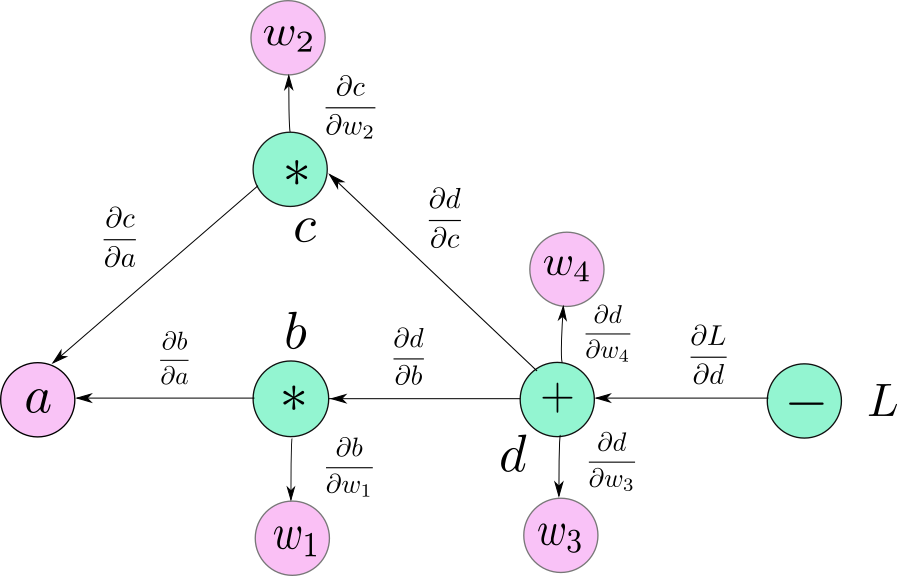$\frac{\partial{L}}{\partial{w_2}} = \frac{\partial{L}}{\partial{d}} * \frac{\partial{d}}{\partial{c}} * \frac{\partial{c}}{\partial{w_2}} \\$

$J = [\frac{\partial{L}}{\partial{w_1}}, \frac{\partial{L}}{\partial{w_2}}, \frac{\partial{L}}{\partial{w_3}}, \frac{\partial{L}}{\partial{w_4}}]$

I可以表示损失函数，y表示输出，x表示中间的数据或者权重参数。中间层可以类比，每一层都可以看做是一个雅克比矩阵，v可能就是一个矩阵了。通过迭代可以求得loss对任意参数的导数。

### 4.2 PyTorch 功能

PyTorch 有两种求导方法。

#### 4.2.1 方法1 backward

    def backward(self, gradient=None, retain_graph=None, create_graph=False, inputs=None):
r"""
Args:
tensor. If it is a tensor, it will be automatically converted
to a Tensor that does not require grad unless create_graph is True.
None values can be specified for scalar Tensors or ones that
don't require grad. If a None value would be acceptable then
this argument is optional.
retain_graph (bool, optional): If False, the graph used to compute
the grads will be freed. Note that in nearly all cases setting
this option to True is not needed and often can be worked around
in a much more efficient way. Defaults to the value of
create_graph.
create_graph (bool, optional): If True, graph of the derivative will
be constructed, allowing to compute higher order derivative
products. Defaults to False.
inputs (sequence of Tensor): Inputs w.r.t. which the gradient will be
accumulated into .grad. All other Tensors will be ignored. If not
provided, the gradient is accumulated into all the leaf Tensors that were
used to compute the attr::tensors. All the provided inputs must be leaf
Tensors.
"""
if has_torch_function_unary(self):
return handle_torch_function(
Tensor.backward,
(self,),
self,
retain_graph=retain_graph,
create_graph=create_graph,
inputs=inputs)



• gradient: 如果张量是非标量（即其数据有多个元素）且需要梯度，则backward函数还需要指定“梯度”。它应该是类型和位置都匹配的张量。
• retain_graph: 如果设置为False，用于计算梯度的图形将被释放。通常在调用backward后，会自动把计算图销毁，如果要想对某个变量重复调用backward，需要将该参数设置为True
• create_graph: 当设置为True的时候可以用来计算更高阶的梯度。
• inputs ：需要计算梯度的张量。如果没有提供，则梯度被累积到所有叶子张量上。

a = torch.tensor(1.0, requires_grad=True)
z = x**3+b
z.backward()


tensor([ 8., 10., 12.])
tensor([147., 192., 243.])


torch.autograd.backward([z], inputs=[a])


import torch

x = torch.randn(2, 2, dtype=torch.double, requires_grad=True)
y = torch.randn(2, 2, dtype=torch.double, requires_grad=True)

def fn():
return x ** 2 + y * x + y ** 2



tensor([[-1.0397, -2.4018],
[-0.5114,  0.2455]], dtype=torch.float64)
tensor([[-0.9240, -2.5764],
[-1.4938,  1.2254]], dtype=torch.float64)


def grad(
outputs: _TensorOrTensors,
inputs: _TensorOrTensors,
retain_graph: Optional[bool] = None,
create_graph: bool = False,
only_inputs: bool = True,
allow_unused: bool = False
) -> Tuple[torch.Tensor, ...]:
r"""Computes and returns the sum of gradients of outputs with respect to
the inputs.

grad_outputs should be a sequence of length matching output
containing the "vector" in Jacobian-vector product, usually the pre-computed
then the gradient can be None).

If only_inputs is True, the function will only return a list of gradients
w.r.t the specified inputs. If it's False, then gradient w.r.t. all remaining
leaves will still be computed, and will be accumulated into their .grad
attribute.

.. note::

If you run any forward ops, create grad_outputs, and/or call grad
in a user-specified CUDA stream context, see
:ref:Stream semantics of backward passes<bwd-cuda-stream-semantics>.

Args:
outputs (sequence of Tensor): outputs of the differentiated function.
inputs (sequence of Tensor): Inputs w.r.t. which the gradient will be
returned (and not accumulated into .grad).
grad_outputs (sequence of Tensor): The "vector" in the Jacobian-vector product.
Usually gradients w.r.t. each output. None values can be specified for scalar
Tensors or ones that don't require grad. If a None value would be acceptable
for all grad_tensors, then this argument is optional. Default: None.
retain_graph (bool, optional): If False, the graph used to compute the grad
will be freed. Note that in nearly all cases setting this option to True
is not needed and often can be worked around in a much more efficient
way. Defaults to the value of create_graph.
create_graph (bool, optional): If True, graph of the derivative will
be constructed, allowing to compute higher order derivative products.
Default: False.
allow_unused (bool, optional): If False, specifying inputs that were not
used when computing outputs (and therefore their grad is always zero)
is an error. Defaults to False.
"""
outputs = (outputs,) if isinstance(outputs, torch.Tensor) else tuple(outputs)
inputs = (inputs,) if isinstance(inputs, torch.Tensor) else tuple(inputs)
overridable_args = outputs + inputs
if has_torch_function(overridable_args):
return handle_torch_function(
overridable_args,
outputs,
inputs,
retain_graph=retain_graph,
create_graph=create_graph,
only_inputs=only_inputs,
allow_unused=allow_unused,
)

if not only_inputs:
warnings.warn("only_inputs argument is deprecated and is ignored now "
"(defaults to True). To accumulate gradient for other "

if retain_graph is None:
retain_graph = create_graph

return Variable._execution_engine.run_backward(


• outputs: 结果节点，微分函数的输出，即需要求导的那个函数。
• inputs: 叶子节点，即返回的梯度，即函数的自变量。
• grad_outputs: Jacobian-vector 积中的向量。
• retain_graph: 如果设置为False，用于计算梯度的图形将被释放。通常在调用backward后，会自动把计算图销毁，如果要想对某个变量重复调用backward，需要将该参数设置为True
• create_graph: 当设置为True的时候可以用来计算更高阶的梯度。
• allow_unused: 默认为False, 即必须要指定input,如果没有指定的话则报错。

import torch

z = x ** 2 + y * x + y ** 2
z.backward(torch.ones(2, 2), create_graph=True)

x_grad = 2 * x + y
y_grad = x + 2 * y

inputs=[x], create_graph=True)



tensor([[ 2.3553, -1.9640],
tensor([[ 1.8319, -2.8185],


### 4.3 模拟印证

#### 4.3.1 原始版本

import torch

y = torch.log(x1) + x1 * x2 - torch.sin(x2)

print('y is :', y)


y is : tensor(11.6521, grad_fn=<SubBackward0>)


#### 4.3.2 前向模式

import torch

v1 = torch.log(x1)
v2 = x1 * x2
v3 = torch.sin(x2)
v4 = v1 + v2
y = v4 - v3

print('v1 is :', v1)
print('v2 is :', v2)
print('v3 is :', v3)
print('v4 is :', v4)
print('y is :', y)

print('dv1 is :', dv1)
print('dv2 is :', dv2)
print('dv3 is :', dv3)
print('dv4 is :', dv4)


v1 is : tensor(0.6931, grad_fn=<LogBackward>)
dv1 is : (tensor(0.5000),)
dv2 is : (tensor(5.),)
dv3 is : (tensor(0.2837),)
dv4 is : (tensor(5.5000), tensor(2.))


#### 4.3.3 反向模式

import torch

v1 = torch.log(x1)
v2 = x1 * x2
v3 = torch.sin(x2)
v4 = v1 + v2
y = v4 - v3

print('v1 is :', v1)
print('v2 is :', v2)
print('v3 is :', v3)
print('y is :', y)

print('dv4 is :', dv4)
print('dv3 is :', dv3)
print('dv2 is :', dv2)
print('dv1 is :', dv1)
print('dv0 is :', dv0)
print('dv21 is :', dv21)
print('dv22 is :', dv22)
print('dv11 is :', dv11)



v1 is : tensor(0.6931, grad_fn=<LogBackward>)
dv4 is : (tensor(1.),)
dv3 is : (tensor(-1.),)
dv2 is : (tensor(1.),)
dv1 is : (tensor(1.),)
dv0 is : (tensor(0.2837),)
dv21 is : (tensor(5.),)
dv22 is : (tensor(2.),)
dv11 is : (tensor(0.5000),)


## 0xFF 参考

https://blog.paperspace.com/pytorch-101-understanding-graphs-and-automatic-differentiation/

Yann LeCun：深度学习已死，可微分编程万岁！

TensorFlow可微编程实践2---自动微分符号体系

https://en.wikipedia.org/wiki/Automatic_differentiation

Pytorch学习2020春-1-线性回归

【深度学习之美01】什么是（机器/深度）学习？

【深度学习之美15】如何感性认识损失函数？

【深度学习之美18】到底什么是梯度？

【深度学习之美21】BP算法详解之前向传播

【深度学习之美22】BP算法详解之链式法则

【深度学习之美23】BP算法详解之反向传播

【深度学习理论】纯公式手推+代码撸——神经网络的反向传播+梯度下降

Automatic Differentiation in Machine Learning: a Survey, https://arxiv.org/pdf/1502.05767.pdf

The Autodiff Cookbook

Automatic Differentiation in Machine Learning: a Survey

PyTorch 的 backward 为什么有一个 grad_variables 参数？

BACKPACK: PACKING MORE INTO BACKPROP

PyTorch自动微分基本原理